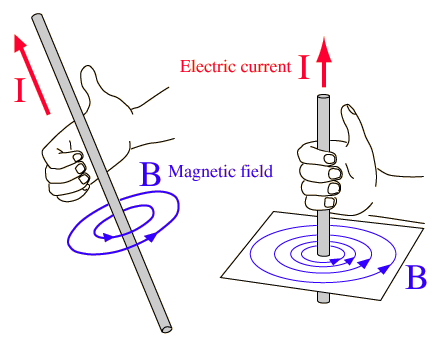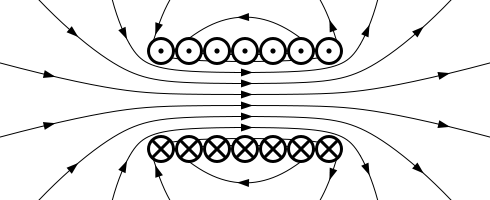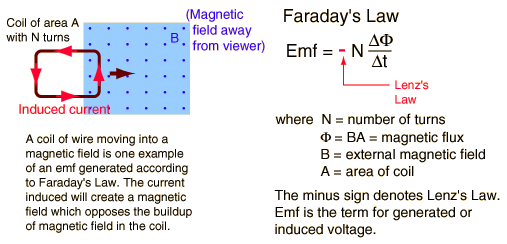Electromagnetism
A simplified explanation for people of all ages
 The demonstration shows a few concepts of electromagnetism in action. First, the solenoid. It is a scientific fact that current running through a wire will create a magnetic field around the wire. The magnetic field is produced tangentially in such a way that it obeys the "right hand rule": Pretend that you are giving a thumbs up. The thumb is the direction of the current, and the way your fingers curl is the direction of the magnetic field.An image depicting the right hand rule. (Source: http://hyperphysics.phy-astr.gsu.edu/hbase/magnetic/magcur.html)          A solenoid produces a magnetic field through this phenomena by focusing these fields in the center by making a circular coil. Applying the right hand rule to a few coils stacked on top of each other and it is apparent that the magnetic field produced by each coil is pointed in the same direction, and therefore add together to produce a stronger magnetic field in the center that is proportional to how many loops of coil the solenoid has. Therefore, the direction of the current of the wire dictates which magnetic polarity is produced on the top or bottom of the solenoid. In the demonstration, I used solenoids with an iron core which is stronger because the iron is made in to a magnet through the magnetic fields produced by the solenoid, while a medium consisting of air does not help proprogate the magnetic field as effectively.An illustration of a cross section of a solenoid. The x on the wire depicts a current going in to the page, while the dot represents a current running in to the page. Source: http://en.wikipedia.org/wiki/Solenoid          Similar to how a running current through a wire produces a magnetic field, a loop of wire running through a magnetic field will produce a current in the loop. This scientific phenomona is classified as "Faraday's Law of Induction". There are two ways in seeing why this happens. The first is that a force is created on the electrons inside the wire when it is moved through a magnetic field. To determine the direction of this force, another right hand rule is used where your hand looks like it is about to give a handshake. The thumb pointing perpendicular to the outstretched fingers is the direction of the velocity, the outstretched fingers are the direction of the magnetic field and the palm is the direction of the force. This force pushes the electrons in that direction, and therefore a current is induced within the wire. The other way is to introduce another concept called the flux, which is the area of the magnetic field that the loop encloses. As the loop moves, more and more of the magnetic field is enclosed by the loop, which increases the flux. The current in the wire is induced in such a way that the magnetic field produced by a current running through a wire counteracts this flux completely.A figure explaining the second way that a current can be induced in a loop. Note that the magnetic field should be x's instead of dots. Source:http://hyperphysics.phy-astr.gsu.edu/hbase/electric/farlaw.html